# Physics - Electromagnetism - Electric potential exercises (part 2)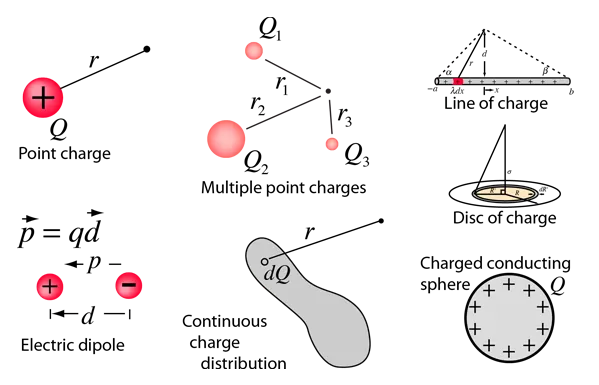Source

## Introduction

Hello it's a me again Drifter Programming!

Today we continue with Electromagnetism to get into more exercises of potential! This is the second part and I highly suggest you to read the first part's post first.

This post will contain applications of potential gradient and more advanced examples of potential in a whole!

All the mathematical equation will be drawn using quicklatex!

So, without further do, let's get straight into it!

There we proved that the electric field and potential are "binded" together using this equation: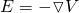which as we said during this post means:

The electric field E is the negative of the gradient (or del) of the potential V.

When the electric field is radial then we mostly use the following: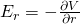So, now that I reminded you of the most important stuff, let's get into how we can use this for calculating the electric field when knowing the potential.

### Potential and electric field of a point charge

The potential in a radial distance r away of a point charge q is given by:The electric field is of course symmetrical and radial and so we can use the second equation we talked about in my small recap.

That way: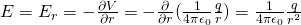The result that we got is exactly the equation that describes an electric field around a point charge that we covered before!

Note that when derivating/differentiating using r the other variables are supposed constant and so remain the same.

The derivative of 1/r is:And that's why the 'minus' disapears from the final result.

### Potential and electric field outside of a charged cylinder

In part 1 we proved that the potential outside of a charged cylinder is given by: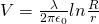where R is the radius of the cylinder and λ the linear charge density.

The electric field of this distribution again only has a radial component, cause else the electric field inside of the cylinder would not be zero, something that interferes with electrostatic!

This means that we can use the "second" equation again and get: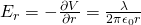which again is the same that we got using Gauss's law and can also calculate with a looooot of math using Coulomb's law!

### Potential and electric field of a charged disk

Last time we proved that the potential of charged disk of radius a and total charge Q, at a distance x away of the center following the axis (which is vertically across to the disk's surface) is given by: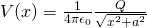The component in the x-axis is:which is the same that we calculated before using Coulomb's law!

Of course in this example the potential doesn't seem to be a function of y or even z, but we can't say that Ey is zero everywhere.

Ey = 0 only at points on the axis-x where y =0.

If we had calculated the more generic form of V for all the points in space then we could find the component y using: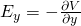and so on...

1. Variation at a cathode tube

In the following: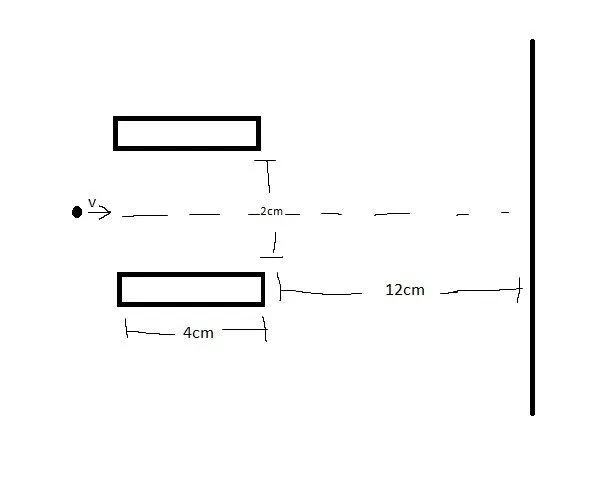an electron is launched with an initial velocity v = 8 x 10^6 m/s  at the center of two conductive plates of a cathode ray tube. The electric field has an upward direction and magnitude E = 6 x 10^3 N/C.

Calculate:

a) How much relative to the axis (center) has the electron moved down when leaving the electric field.

b) The angle in which the electron moves relative to the axis after leaving the plates.

c) The point of impact on the fluorescent display (the distance relative to the axis).

Suppose that there is no gravity!

a)

The electric field "goes" upwards, but because the electron is negatively charged an opposite force F acts on it with magnitude F = -eE.

That way the acceleration using Newton's 2nd law is: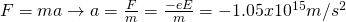of course the mass and charge of an electron are known and approximately: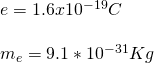Those are the values I used for my calculations...

Either way, because the force is constant the motion is similar to (or exactly) a projectile motion that we covered in Classical mechanics.

This means that the point of impact can be found easily using:
Oops...we now need to calculate the time needed to leave the electric field.

This is of course also easy, cause we have a constant velocity and know the distance.

The time needed is: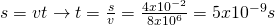And so the distance traveled is: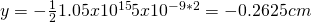b)

The angle or the tangent of the angle can be found using the two velocities.

The x-velocity is constant...

The y-velocity when leaving the electric field is: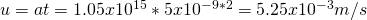And so the tangent of the angle is: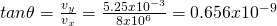c)

The point of impact can be easily calculated by finding the time needed to travel from the end of the plates to the display and supposing the velocity constant downwards constant and equal to the lastly calculated value.

That way the distance will be:where y0 the distance away that we had when leaving the plates and the acceleration of course now zero, cause no force acts anymore.

I will not calculate this, try doing this on your own...

Also, when having exponentials I tend to do things wrong and so just keep the way of thinking, the results might be false!

2. Coaxial Cylinders

Suppose we have two cylinders with radius a and b (a<b) and charge densities +λ and -λ respectively and so the small cylinder is positively charged, whilst the other one is negatively charged.

Calculate the potential V(r) for the following cases:

i) r<a (inside of the "positive" cylinder)

ii) a<r<b (in the area between)

iii) r>b (inside of the "negative" cylinder)

During this post we found the electric field and last time also the potential for an cylinder.

The total potential is of course some sum between the potentials of the two cylinders.

The potential can't be supposed zero at infinity and so we will suppose V = 0 when r = b.

i)

The potential difference when r<a is:The result is similar to what we proved last time for disks that was:Here something even more interesting...

The electric field again is radial and so we can partially differentiate the upper-equation to get the electric field which is:A very simple equation that doesn't contain the Coulomb constant anymore!

This equation is of course also true even if the cylinder outside had no charge, cause the electric field again stays the same!

Remember that we have to set a point of reference where V = 0 and so by "notating" it as b we get the same result!

ii)

For in-between values we have to find the potential difference Vrb which gives us: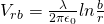iii)

Because of our assumption (V=0 at r =b) and because the electric field is zero outside of the second cylinder we know that the potential difference of course is also V = 0.

Intro

Physics Introduction -> what is physics?, Models, Measuring

Vector Math and Operations -> Vector mathematics and operations (actually mathematical analysis, but I don't got into that before-hand :P)

Classical Mechanics

Velocity and acceleration in a rectlinear motion -> velocity, accelaration and averages of those

Rectlinear motion with constant accelaration and free falling -> const accelaration motion and free fall

Rectlinear motion with variable acceleration and velocity relativity -> integrations to calculate pos and velocity, relative velocity

Rectlinear motion exercises -> examples and tasks in rectlinear motion

Position, velocity and acceleration vectors in a plane motion -> position, velocity and accelaration in plane motion

Projectile motion as a plane motion -> missile/bullet motion as a plane motion

Smooth Circular motion -> smooth circular motion theory

Plane motion exercises -> examples and tasks in plane motions

Force and Newton's first law -> force, 1st law

Mass and Newton's second law -> mass, 2nd law

Newton's 3rd law and mass vs weight -> mass vs weight, 3rd law, friction

Applying Newton's Laws -> free-body diagram, point equilibrium and 2nd law applications

Contact forces and friction -> contact force, friction

Dynamics of Circular motion -> circular motion dynamics, applications

Object equilibrium and 2nd law application examples -> examples of object equilibrium and 2nd law applications

Contact force and friction examples -> exercises in force and friction

Circular dynamic and vertical circle motion examples -> exercises in circular dynamics

Electromagnetism

Getting into Electromagnetism -> electromagnetim, electric charge, conductors, insulators, quantization

Coulomb's law with examples -> Coulomb's law, superposition principle, Coulomb constant, how to solve problems, examples

Electric fields and field lines -> Electric fields, Solving problems around Electric fields and field lines

Electric dipoles -> Electric dipole, torque, potential and field

Electric charge and field Exercises -> examples in electric charges and fields

Electric flux and Gauss's law -> Electric flux, Gauss's law

Applications of Gauss's law (part 1) -> applying Gauss's law, Gauss applications

Applications of Gauss's law (part 2) -> more Gauss applications

Electric flux exercises -> examples in electric flux and Gauss's law

Electric potential energy -> explanation of work-energy, electric potential energy

Calculating electric potentials -> more stuff about potential energy, potential, calculating potentials

Millikan's Oil Drop Experiment -> Millikan's experiment, electronvolt

Cathode ray tubes explained using electric potential -> cathode ray tube explanation

Electric potential exercises (part 1) -> applications of potential

And this is actually it for today!

From next time we will start getting into electronic circuit stuff, starting with capacitors!

Bye!

H2
H3
H4
3 columns
2 columns
1 column